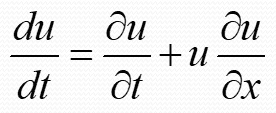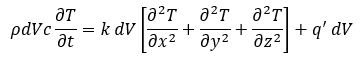# How do you know when to partially differentiate?

• I
Zheng_
The equation of flow acceleration iswhere du/dt is the global acceleration, and ∂u/∂t and u ∂u/∂x are local and convective acceleration respectively.
And the heat conduction equation iswhich is heat stored = net heat flux + heat generated.

My question is: why does the ∂T/∂t part in the heat conduction equation is partially differentiated, while the du/dt part in the flow acceleration isn't?

#### Attachments

Homework Helper
Gold Member
2022 Award
In the first equation, u is a function of x and t. At any instant (i.e. t constant) there is a velocity at each position x, and a gradient in the velocity field as we consider small changes in x. Likewise, at any location, we can observe particles at that point over time and note how the local velocity changes there.
But if we fix on a particle at some (x,t), not only is it accelerating because of the local acceleration, it may also be accelerating because it is moving to somewhere with a different local velocity. We are interested in how the velocity of this particle changes over time.
It can help to visualise this as a surface, where the horizontal coordinates are x and t, and the height is u. The slope in the x direction shows how much faster or slower nearby particles are moving at an instant, while the slope in the t direction shows how the velocity of particles observed at the fixed location changes with time.
The surface has a tangent plane at this location and time. The slope in any given direction from there can be deduced from the two coordinate slopes.
If we follow a particle from this location, it tracks in a "direction" given by its current speed, u. After time Δt its speed will have changed by ##+\Delta t\frac{\partial u}{\partial t}## by virtue of the acceleration (slope) ##\frac{\partial u}{\partial t}## in the time direction, and by ##\Delta x\frac{\partial u}{\partial x}## by virtue of the slope ##\frac{\partial u}{\partial x}## in the x direction. But we know that ##\Delta x=u\Delta t##, so ##\Delta u=u\Delta t\frac{\partial u}{\partial x}+\Delta t\frac{\partial u}{\partial t}##. Hence the equation you posted.
It is defining u as the velocity of a given particle that is key.

In the temperature equation, we are not fixing on some "packet" of heat that moves around. Instead, ##T=T(\vec x, t)##, where the position and time are independent. There is no ##\frac{dT}{dt}## to be considered. Of course, we could invent a bug that crawls around according to some ##\vec x=\vec x(t)## and ask how it experiences changing temperatures. Then we would have ##\frac{dT_{bug}}{dt}=\frac{\partial T}{\partial t}+\dot{\vec x}.\nabla T##.

•Zheng_